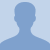# Rotational Motion: Angular AccelerationThis lesson will define angular acceleration and explain how to solve for a basic angular acceleration. In relation to kinematics and angular velocity. The change in angular velocity over the change in time and the formulas... This lesson will define angular acceleration and explain how to solve for a basic angular acceleration. In relation to kinematics and angular velocity. The change in angular velocity over the change in time and the formulas required to solve for angular acceleration will be looked at in depth in this lesson.
More... Collapse
11 Views

## Comments (0)

Please log in to post comments.